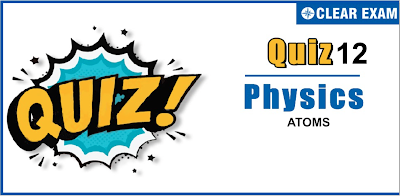## ATOMS QUIZ-12

JEE Advanced Physics Syllabus can be referred by the IIT aspirants to get a detailed list of all topics that are important in cracking the entrance examination. JEE Advanced syllabus for Physics has been designed in such a way that it offers very practical and application-based learning to further make it easier for students to understand every concept or topic by correlating it with day-to-day experiences. In comparison to the other two subjects, the syllabus of JEE Advanced for physics is developed in such a way so as to test the deep understanding and application of concepts.

Q1. How many revolutions does an electron complete in one second in the first orbit of hydrogen atom?
•  6.62×10^15
•  100
•  1000
•  1
Q2. Angular momentum (L) and radius (r) of a hydrogen atom are related as
•  Lr= constant
•  Lr^2= constant
•  Lr^4= constant
•  None of these
Q3. The total energy of an electron in the ground state of hydrogen atom is -13.6 eV. The potential energy of an electron is the ground state of Li^(2+) ion will be
•   122.4 eV
•  -122.4 eV
•  244.8 eV
•  -244.8 eV

Q4. If elements with principal quantum number n>4 were not allowed in nature, the number of possible elements would have been
•  32
•  60
•  64
•  4
Q5. An electron collides with a hydrogen atom in its ground state and excites it to n=3. The energy given to hydrogen atom in this inelastic collision is [Neglect the recoiling of hydrogen atom]
•  10.2 eV
•  12.1 eV
•  12.5 eV
•  None of these
Q6. In terms of Rydberg constant R, the shortest wavelength in Balmer series of hydrogen atom spectrum will have wavelength
•  1/R
•  4/R
•  3/2R
•  9/R
Q7. An atom emits a spectral line of wavelength λ when an electron makes a transition between levels of energy E_1 and E_2. Which expression correctly relates λ,E_1 and E_2?
•  λ=hc/(E_1+E_2 )
•  λ=2hc/(E_1+E_2 )
•  λ=2hc/(E_1-E_2 )
•  λ=hc/(E_1-E_2 )
Q8. In which of the following transitions will the wavelength be minimum?
•  n_1=5 to n_2=4
•  n_1=4 to n_2=3
•  n_1=3 to n_2=2
•  n_1=2 to n_2=1
Q9. An electron in a Bohr orbit of hydrogen atom with the quantum number n_2 has an angular momentum 4.2176×10^(-34) kg m^2 s^(-2). If the electron drops from this level to the next lower level, the wavelength of this line is
•  18 nm
•  187.6 pm
•  1876 Å
•  1.876×10^4 Å
Q10. In hydrogen and hydrogen-like atoms, the ratio of E_4n-E_2n and E_2n-E_n varies with atomic number z and principal quantum number n as
•  z^2/n^2
•  z^4/n^4
•  z/n
• None of these#### Written by: AUTHORNAME

AUTHORDESCRIPTION## Want to know more

Please fill in the details below:

## Latest NEET Articles\$type=three\$c=3\$author=hide\$comment=hide\$rm=hide\$date=hide\$snippet=hide

Name

ltr
item
BEST NEET COACHING CENTER | BEST IIT JEE COACHING INSTITUTE | BEST NEET, IIT JEE COACHING INSTITUTE: Atoms - Quiz 12
Atoms - Quiz 12
https://1.bp.blogspot.com/-XPC9X40A9ZA/YOcQca8_T9I/AAAAAAAAUpo/7Sn08OkRdSISlCW-Hsds79uiDGPlVEcCQCLcBGAsYHQ/s400/12.png
https://1.bp.blogspot.com/-XPC9X40A9ZA/YOcQca8_T9I/AAAAAAAAUpo/7Sn08OkRdSISlCW-Hsds79uiDGPlVEcCQCLcBGAsYHQ/s72-c/12.png
BEST NEET COACHING CENTER | BEST IIT JEE COACHING INSTITUTE | BEST NEET, IIT JEE COACHING INSTITUTE
https://www.cleariitmedical.com/2021/07/atoms-quiz-12.html
https://www.cleariitmedical.com/
https://www.cleariitmedical.com/
https://www.cleariitmedical.com/2021/07/atoms-quiz-12.html
true
7783647550433378923
UTF-8